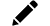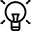﻿# Tiles Calculator – Calculate How Much Tiles You Will Need?

Tile Calculator- a tool used to get an exact number of tiles to be installed in your space. This tool calculates the number of tile boxes required in order to completely cover the total sq. feet area. Also, it will provide you with the number of tiles in each box.

Tile Calculator

Here are the details for the tiles of your choice.

Wall Floor

We recommend accounting for an additional 10% wastage due to any accidental damage that might occur or when tiles have to be cut around the edges of the room.

%Wall Floor
Yes No
Wall 1If there are large obstructions in the wall such as doors and windows, multiply the width by the height of the obstruction then subtract that from the total area you need to tile.

## Calculating the Amount of Tiles Needed

When it comes to a home renovation or construction project that involves tiling, it is important to calculate the amount of tiles you'll need in order to complete the job. This will help you make sure you have enough tiles, and that you're not wasting money on tiles that you don't need. In this article, we will cover the steps for calculating the amount of tiles you'll need for your project.

### Step 1: Measure the space where the tiles will be installed:

The first step in calculating the amount of tiles you’ll need is to measure the space where the tiles will be installed. Be sure to measure the width and length of the space, as well as the height if you’re installing tiles on a wall. it is important to be as accurate as possible when measuring, as this will affect the final calculation.

### Step 2: Calculate the square footage of the space:

Once you have the dimensions of the space, the next step is to calculate the square footage. This is simply the width multiplied by the length of the space. For example, if the space you are tiling is 10 feet wide and 12 feet long, the square footage would be 10 x 12 = 120 square feet.

### Step 3: Determine the size of the tiles you’ll be using:

Next, you’ll need to determine the size of the tiles you'll be using. The size of the tiles will affect the final calculation, as larger tiles will cover more area than smaller tiles. Be sure to measure the width and length of each tile, and write down the dimensions.

### Step 4: Calculate the number of tiles needed:

Once you have the square footage of the space and the size of the tiles, you can calculate the number of tiles you'll need. This is simply the square footage of the space divided by the area of each tile. For example, if the square footage of the space is 120 square feet and each tile is 12 inches by 12 inches, the calculation would be 120 square feet / (12 inches x 12 inches) = 120 square feet / 144 square inches = 0.83 tiles.

### Step 5: Add a buffer for waste and cuts:

It’s important to remember that when installing tiles, there will be some waste and cuts. In order to account for this, you'll need to add a buffer to the final calculation.As a general rule, you should add 10% to the final calculation to account for waste and cuts. So, if the calculation in step 4 resulted in 0.83 tiles, you would need to add 0.83 x 10% = 0.08 tiles to account for waste and cuts.

## FAQs:

### How to calculate tiles needed for the floor or wall?

Once you have selected the tiles of your choice, you need to measure the floor or wall where these tiles will be installed. Now divide the total sq. feet of the space by the size of the tile. This will give you the exact number of tiles to be installed.

### How the tile calculator works, and how to calculate a tile's square footage?

Tile calculator gives you the total number of boxes required to cover the total sq. feet of the area. First of all, multiply the length and width of the tile in feet. This will give you the tile’s square footage. Then simply add this number to the tile calculator, and it will provide you with the number of boxes of tiles required.

### How to calculate the area?

The simple way to calculate the area is to measure the length and width of the space and then multiply them. The answer will be the exact square footage of the area.

### How many extra tiles should I buy?

When it comes to tile projects, you should get at least 10% extra tiles in order to account for wastage and tiles broken, cracked or chipped in transit or due to mishandling. Also, in the future, if there is any breakage, you can use an extra piece of tile to mend it.

### How do I calculate how much flooring I need?

To ensure the right amount of flooring, you need to first make sure you measure the area properly. Calculate the total sq. feet of the room and divide it by the size of the tile. This will give you the exact calculation of the flooring required.

### How many tiles cover 1 sqm?

It depends on the size of the tile and how many tiles will be required to cover 1 sq m. Let’s say if there is a 600x600mm tile; then you would require around 3 tiles to cover 1 sq m. Just like that you can calculate the number of tiles according to the size of the tile.

### How many tiles do I need for 1000 square feet?

If you want to cover a space with a 1000 sq. feet area then you have to divide it by the size of the tile. The larger the size of the tile, the less number of tile pieces required and vice versa.

### How much does it cost to tile a 10X10 bathroom?

Multiply the total square feet of the area by the labour rate to get the exact cost to tile a bathroom. Also, you can add the total money spent on the tile buying with the labour rate to find out the cost to tile a 10x10 bathroom.

### Can you tile in a day?

Yes, if you have a small space, you can tile a space in a day. But if you have a spacious room to be tiled, it may take a day or two. If you get it done by professionals then they might take less time to tile a room.

### What is the labour cost for laying a tile?

The labour cost for laying a tile in India is generally Rs. 30 to Rs. 40 per sq. feet. It actually depends on the type of tile as well. If there is a ceramic tile, then the labour cost will be a little less in comparison to vitrified tiles. Installing larger tiles is marginally more expensive than installing smaller tiles. If you want to make fancy designs using multiple tiles your labour charges may go up even more.

### Is it cheaper to tile over tile?

Yes, it reduces the time wastage as you don’t have to take off the original tiles. Also, the labour cost of first removing the original tiles then installing new ones increases the total cost.

### Which is cheaper: ceramic tile or porcelain tile?

Ceramic and porcelain are comparatively cheaper than vitrified tiles. However when it comes to the price of ceramic and porcelain tiles, the cheaper one is ceramic tile. Ceramic and porcelain tiles are made with a clay mixture, but the porcelain tiles are fired at a higher temperature. This makes ceramic tiles cheaper than porcelain tiles.

### How much tile do I need for 20x20?

The total area calculation is 20x20=400 sq. feet. Divide this figure with the size of the tile you want to be installed. This will give you the exact number of tiles required for your space.

### Is it cheaper to carpet or tile?

Tiles are of low-maintenance however the carpet would require cleaning very frequently. This keeps on increasing the cost and the time spent on the carpet maintenance. Therefore, tiles are cheaper than carpet and a good-to-go option for most of the people.